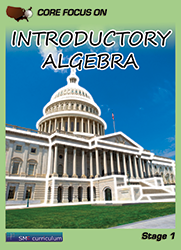#### Introductory Algebra

In this course students apply and extend previous understandings of arithmetic to algebraic expressions. They reason about and solve one-variable equations and inequalities. Students apply and extend previous understandings of numbers to the system of rational numbers. At the end of the course, they learn to represent and analyze quantitative relationships between dependent and independent variables.

### Textbook & Stage I Series Pricing

1 FREE Teacher Edition(TE) and Teacher Resource Binder(TR) per 40 student texts ordered!